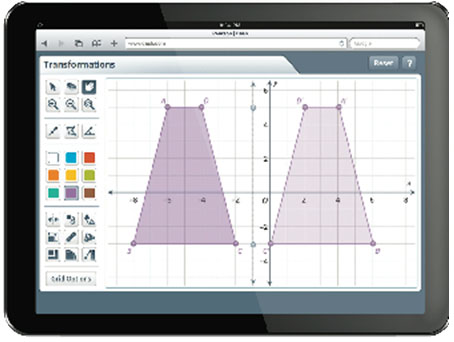Display Accessibility Tools

# Technology Tools

### Digital Math Tools

Digital math tools are available via a menu on Student Place and Teacher Place. Students can use the digital tools at any time during their work on Problems or exercises. The teacher support will suggest when teachers may want to use a particular tool to facilitate the Problem or during the Summarize. For example, the integer chips tool can be used instead of physical chips during the Problems introducing integer operations.

The following Digital Math Tools are available on Student Place and Teacher Place:

• 3D Geometry
• Coordinate Grapher
• Expression Calculator
• Geoboard
• Number Charts
• Pattern Blocks
• Algebra Tiles
• Data and Graphs
• Fraction Shapes
• Integer Chips
• Number Line
• Probability

### Student Activities### MathXL

MathXL for School, developed by Savvas, is used in CMP3 to provide additional skills practice online, thereby replacing the CMP2 Additional Practice and Skills Workbooks. A link to MathXL is available via the Links menu in Student Place and Teacher Place. Teachers can assign skills practice by Unit to individuals or groups of students within MathXL. For each Unit, teachers also have the option of assigning students a short readiness test or a longer skills assessment at the conclusion of the Unit, which can inform the practice assignments. Thus, the student is only assigned practice on the skills the student has not yet mastered. MathXL is intended as a supplement and not as a replacement for the ACE in CMP3.

### Calculators

Connected Mathematics (CMP) was developed with the belief that calculators should be available to students, and that students should know when and how to use them. Graphing calculators with table and statistical-display capabilities or similar digital tools, are used in all three grades.

In grade 6, students use calculators to simplify complicated calculations and explore patterns in computations. In grades 7 and 8, graphing calculators are used to investigate functions and as a tool for solving problems. Students use graphing calculators to explore the shape and features of graphs of linear, exponential, and quadratic functions as well as the patterns of change in the tables of such functions. Furthermore, in addition to using symbolic solution methods, students can use graphing-calculator tables and graphs to solve equations, as well as to display data and calculate statistical measures.